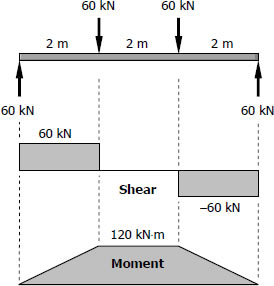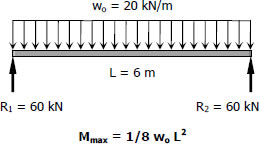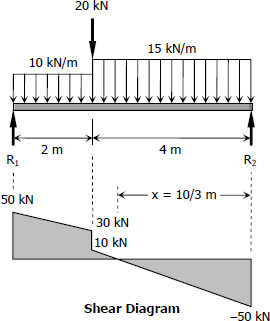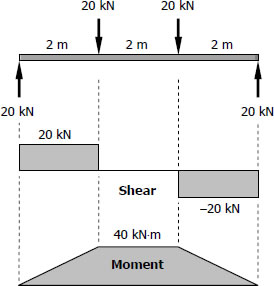# economic section

## For Girders (G - 2)$S_{required} = \dfrac{M}{f_b} = \dfrac{120(1000^2)}{120}$
$S_{required} = 1000 \times 10^3 \, \text{mm}^3$

## For Beams (B - 3)$M_{max} = \frac{1}{8}(20)(62)$
$M_{max} = 90 \, \text{kN}\cdot\text{m}$

$S_{required} = \dfrac{M_{max}}{f_b} = \dfrac{90(1000^2)}{120}$
$S_{required} = 750 \times 10^3 \, \text{mm}^3$

## For Beams (B - 2)$\Sigma M_{R2} = 0$
$6R_1 = 20(4) + 10(2)(5) + 15(4)(2)$
$R_1 = 50 \, \text{kN}$

$\Sigma M_{R1} = 0$
$6R_2 = 20(2) + 10(2)(1) + 15(4)(4)$
$R_2 = 50 \, \text{kN}$

## For Girder (G - 1) | Solution to Problem 542

For Girder (G - 1)$S_{live-load} = \dfrac{M}{f_b} = \dfrac{40(1000^2)}{120}$
$S_{live-load} = 333.33 \times 10^3 \, \text{mm}^3$